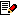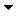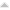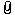Coordinate Frame conversion of coordinatesFrom: bemfarmer 11 Mar 2012  (1 of 6)// Michael, this is a Request for information on coordinate frame, and how are coordinates // converted back and forth with the "world coordinate frame?" var vM = moi.vectorMath; // P1 is a point in world coordinates, which is to be the temporary origin in coordinate frame. // P2 to be a suitable point for creating the frame x axis. // P3 to be a suitable point for creating the frame y axis. // The next 4 lines are correct, from Mirror script: var FrameOrigin = vM.createPoint(P1.x, P1.y, P1.z); var FrameXAxis = vM.createPoint(P2.x, P2.y, P2.z); var FrameYAxis = vM.createPoint(P3.x, P3.y, P3.z); var catFrame = vM.createFrame( FrameOrigin, FrameXAxis, FrameYAxis ); // The first question is, given points P4 and P5, in world coordinates, will the // following code create points Pt4 and Pt5 in catFrame coordinates? var Pt4 = moi.coordinateFrame.evaluate.catFrame(P4.x, P4.y, P4.z); var Pt5 = moi.coordinateFrame.evaluate.catFrame(P5.x, P5.y, P5.z;) // The second question is, having calculated point Pt6, the vertex of parametric catenary // curve between Pt4 and Pt5, using cosh, which I already know how to do, // will the following code convert point Pt6 back into world coordinates? var x = moi.coodinateFrame.distancex(catFrame.Pt6); var y = moi.coodinateFrame.distancey(catFrame.Pt6); var z = moi.coodinateFrame.distancez(catFrame.Pt6); var vertexPt6 = vM.createPoint( x, y, z ); // (next, point vertexPt6 will then become the new origin in a new coordinate frame...) Thank you.EDITED: 2 Apr 2015 by BEMFARMERReply MoreFrom: Michael Gibson 11 Mar 2012  (2 of 6)5000.2 In reply to 5000.1 Hi Brian, > // P1 is a point in world coordinates, which is to be the temporary origin in coordinate frame. > // P2 to be a suitable point for creating the frame x axis. > // P3 to be a suitable point for creating the frame y axis. > // The next 4 lines are correct, from Mirror script: > var FrameOrigin = vM.createPoint(P1.x, P1.y, P1.z); > var FrameXAxis = vM.createPoint(P2.x, P2.y, P2.z); > var FrameYAxis = vM.createPoint(P3.x, P3.y, P3.z); > var catFrame = vM.createFrame( FrameOrigin, FrameXAxis, FrameYAxis ); Which one is the "Mirror script" ? One note is that here P2 and P3 should actually be vectors - meaning that they represent an oriented direction and not a point location. The way a coordinate frame works is that there is one point for its origin and then 3 vector directions for x, y, and z axes. Often you'll just give it the x and y axis directions because the z axis can be calculated from those automatically by doing a cross product. > // The first question is, given points P4 and P5, in world coordinates, will the > // following code create points Pt4 and Pt5 in catFrame coordinates? > > var Pt4 = moi.coordinateFrame.evaluate.catFrame(P4.x, P4.y, P4.z); > var Pt5 = moi.coordinateFrame.evaluate.catFrame(P5.x, P5.y, P5.z;) The syntax here does not quite look correct - there isn't any coordinateFrame member function off of the root moi object, so doing: moi.coordinateFrame isn't going to do anything. I think you want to call something on catFrame directly, so more like: catFrame.evaluate( x, y, z ); But the .evaluate() method on a coordinate frame is for taking an x,y,z point that is in that coordinate system and converting into a world coordinate point. It basically starts at the frame's origin, then goes along the x axis direction of the frame by the x value that you pass in, and then the same for y and z values. So if for example you passed in just evaluate(0,0,0) that would give you back just the origin point. if you passed evalute(1,0,0) that would give back a point that was 1 unit away from the frame's origin in the x axis direction. If you want to go the reverse way of getting a world coordinate point in terms of the frame's coordinate system then you would want to use the distance methods, you can do this: var x = frame.distancex( pt ); var y = frame.distancey( pt ); var z = frame.distancez( pt ); And then that x,y,z point will be a point in that local coordinate system. Each of those calls returns the distance that the passed in point is located at along each frame axis direction, basically a plane that is formed from the origin point and the axis direction. > // The second question is, having calculated point Pt6, the vertex of parametric catenary > // curve between Pt4 and Pt5, using cosh, which I already know how to do, > // will the following code convert point Pt6 back into world coordinates? > > > var x = moi.coodinateFrame.distancex(catFrame.Pt6); > var y = moi.coodinateFrame.distancey(catFrame.Pt6); > var z = moi.coodinateFrame.distancez(catFrame.Pt6); > var vertexPt6 = vM.createPoint( x, y, z ); So if I understand properly, you've got the reverse thing for this part - if you have an x,y,z that is in the local coordinate system of the frame, use the .evaluate() method on the frame to convert to a world coordinate value. Hope this helps, please let me know if it does not make sense. - MichaelReply MoreFrom: bemfarmer 12 Mar 2012  (3 of 6)5000.3 In reply to 5000.2 Thank you very much Michael, for your response. It is very understandable, and useable. :-) (The mirror script is the official moi script in its command subdirectory.) So the x-axis and y-axis direction vectors used to create the new frame would be (or could be) in world coordinate frame. A new y-axis, parallel to the world coordinate y-axis would have the same vector direction, (0,1,0). The new x-axis vector direction can be calculated from the two points, mathematically, or with vectorMath... EDITED: 12 Mar 2012 by BEMFARMERReply MoreFrom: Michael Gibson 12 Mar 2012  (4 of 6)5000.4 In reply to 5000.3 Hi Brian, > So the x-axis and y-axis direction vectors used to create the > new frame would be (or could be) in world coordinate frame. Yup, normally those direction vectors are in world coordinates. The origin is also in world coordinates - the whole package of data gives the position and orientation of the frame's coordinate system. > A new y-axis, parallel to the world coordinate y-axis would > have the same vector direction, (0,1,0). Yup that's correct. > The new x-axis vector direction can be calculated from > the two points, mathematically, or with vectorMath... Yup, but the x-axis vector should be one that is at a 90 degree angle to the y axis vector. But it can be pivoted around the x-axis, like for example here in red are different possible x-axis lines that would work with the given green y axis:- Michael Attachments:brian_axis_pivot.jpgReply MoreFrom: bemfarmer 12 Mar 2012  (5 of 6)5000.5 In reply to 5000.4 Thank you Michael. Should have enough now to finish a catenary curve script hanging from two points in 3D, even with different z values, with gravity in the -y direction. There is plenty of code on the internet. Yes, the new x-axis "rotates" about the new y-axis, to "zero out" the z values. EDITED: 12 Mar 2012 by BEMFARMERReply MoreFrom: bemfarmer 20 Mar 2012  (6 of 6)Just figured out that a minus sign "-" is not the same as a dash "–". So cut and paste of formulas with dashes for minus signs, from Microsoft Word to Notepad++, creates parse errors in javascript.Reply MoreReply to All